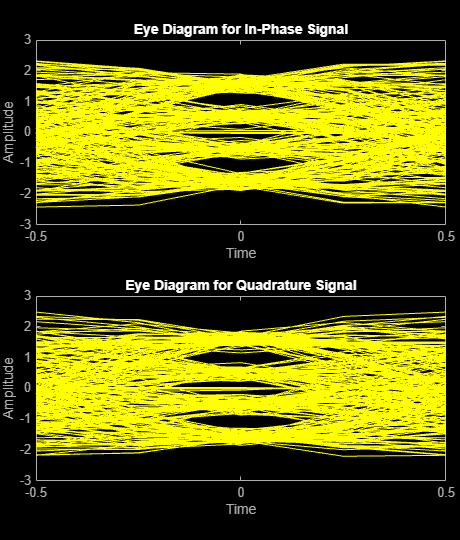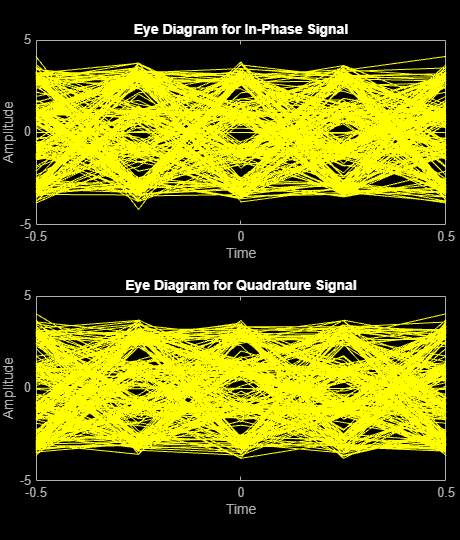# Pulse Shaping Using a Raised Cosine Filter

Filter a 16-QAM signal using a pair of square root raised cosine matched filters. Plot the eye diagram and scatter plot of the signal. After passing the signal through an AWGN channel, calculate the number of bit errors.

Set the simulation parameters.

```M = 16; % Modulation order bps = log2(M); % Bits/symbol n = 20000; % Transmitted bits sps = 4; % Samples per symbol EbNo = 10; % Eb/No (dB)```

Set the filter parameters.

```span = 10; % Filter span in symbols rolloff = 0.25; % Rolloff factor```

Create the raised cosine transmit and receive filters using the previously defined parameters.

```txfilter = comm.RaisedCosineTransmitFilter( ... RolloffFactor=rolloff, ... FilterSpanInSymbols=span, ... OutputSamplesPerSymbol=sps); rxfilter = comm.RaisedCosineReceiveFilter( ... RolloffFactor=rolloff, ... FilterSpanInSymbols=span, ... InputSamplesPerSymbol=sps, ... DecimationFactor=sps);```

Plot the impulse response of the raised cosine transmit filter object `txFilter`.

`fvtool(txfilter,Analysis="impulse")`Calculate the delay through the matched filters. The group delay is half of the filter span through one filter and is, therefore, equal to the filter span for both filters. Multiply by the number of bits per symbol to get the delay in bits.

`filtDelay = bps*span;`

Create an error rate counter System object™. Set the `ReceiveDelay` property to account for the delay through the matched filters.

`errorRate = comm.ErrorRate(ReceiveDelay=filtDelay);`

Generate binary data.

`x = randi([0 1],n,1);`

Modulate the data.

`modSig = qammod(x,M,InputType="bit");`

Filter the modulated signal.

`txSig = txfilter(modSig);`

Plot the eye diagram of the first 1000 samples.

`eyediagram(txSig(1:1000),sps)`Calculate the signal-to-noise ratio (SNR) in dB given `EbNo`. Pass the transmitted signal through the AWGN channel using the `awgn` function.

```SNR = EbNo + 10*log10(bps) - 10*log10(sps); noisySig = awgn(txSig,SNR,"measured");```

Filter the noisy signal and display its scatter plot.

```rxSig = rxfilter(noisySig); scatterplot(rxSig)```Demodulate the filtered signal and calculate the error statistics. The delay through the filters is accounted for by the `ReceiveDelay` property in `errorRate`.

```z = qamdemod(rxSig,M,OutputType="bit"); errStat = errorRate(x,z); fprintf('\nBER = %5.2e\nBit Errors = %d\nBits Transmitted = %d\n',... errStat)```
```BER = 1.15e-03 Bit Errors = 23 Bits Transmitted = 19960 ```